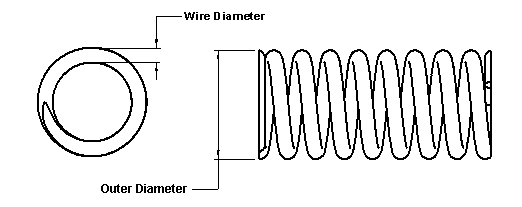Compression Spring Constant Equation and Calculator

Spring Design Menu | Spring Suppliers and Manufacturers

This calculator will determine the spring rate constant related to a compression spring from basic geometry and material data input. Default units are shown in inches, etc however SI (metric) can be used.This equation will determine (estimate) the Spring Rate (k) of a compression spring. Spring rate is best determined by test and measurement techniques.

Open Compression Spring Constant (k) Calculator
(This calculator requires a java - enabled browser)

Equation:

k = Gd4/[8nD3]

Where:

k = constant, pounds of load per inch of deflection
G = modulus of rigidity of spring material, pounds per square inch
d = wire diameter, inches
n = number of active coils, which is the number of coils subjected to flexure (always less than the total number of coils)
D = mean coil diameter, inches = Outer Diameter - Wire Diameter

G is calculated from the spring materials Elastic modulus and Poisson Ratio n

G = E/(2(1 + v)

The pitch of the spring coil can be found by

Cp = Lf / nc

Where:

Lf = Uncompressed length of spring
nc = Number of coils

The angle of the spring coil relativeto the mounting base is found:

f = atan [ Cp / p D ]

Compression Spring Design SuggestionsMembership Register | LoginHomeEngineering Book StoreEngineering ForumExcel App. DownloadsOnline Books & ManualsEngineering NewsEngineering VideosEngineering CalculatorsEngineering ToolboxGD&T Training Geometric Dimensioning TolerancingDFM DFA TrainingTraining Online EngineeringAdvertising CenterCopyright Notice